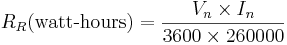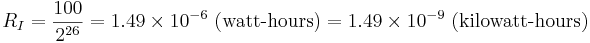## Overview

The WattNode® BACnet and Modbus meters (WNC series) measures energy once per second in raw units. The raw measurements are then scaled based on the meter’s calibration, the nominal line voltage, and the current transformer (CT) rated amps. Finally, the result is added to a 64 bit energy accumulator. When an energy reading is requested from a meter over the network, the energy value in the 64 bit accumulator is scaled appropriately and reported over the network. Essentially, there are three different steps, each with different native energy resolutions.

Also important, as with all instruments, the WattNode measurements have noise as well as systematic errors. Over a short time period (one to five seconds), the energy measurement noise can be noticeable. Over longer time periods, the noise will be integrated out, but systematic errors will remain, such as errors due to imperfect calibration, drift, temperature variations, and current transformer phase angle errors.

## Raw Measurement Resolution

At full-scale power (nominal line Vac, rated CT amps, and unity power factor), the raw energy readings will report approximately 260,000 counts per second, resulting in the following equation for raw resolution:$R_R mbox{(watt-hours)} = frac{V_n times I_n}{3600 times 260000}$
The following table shows the nominal Vac values for different WattNode BACnet and Modbus models and the corresponding raw reading resolution using the smallest (5 amp) and largest (6000 amp) current transformers we sell.

Model Vn
Nominal VAC
5A CT – RR
Raw Resolution
in Watt-hours
6000A CT – RR
Raw Resolution
in Watt-hours
WNC-3Y-208-(BN or MB) 120 6.4 x 10-7 7.9 x 10-4
WNC-3Y-400-(BN or MB) 230 1.2 x 10-6 1.5 x 10-3
WNC-3Y-480-(BN or MB) 277 1.5 x 10-6 1.8 x 10-3
WNC-3Y-600-(BN or MB) 347 1.9 x 10-6 2.2 x 10-3
WNC-3D-240-(BN or MB) 120 6.4 x 10-7 7.7 x 10-4
WNC-3D-400-(BN or MB) 230 1.3 x 10-6 1.5 x 10-3
WNC-3D-480-(BN or MB) 277 1.5 x 10-6 1.8 x 10-3

Note: to convert these into kWh units, divide by 1000.

## Internal Accumulator Resolution

After calibrating and scaling, the energy is added to a 64 bit accumulator register once per second. This register has the following internal resolution:$R_I = frac{100}{2^{26}} = 1.49 times 10^{-6} mbox{ (watt-hours)} = 1.49 times 10^{-9} mbox{ (kilowatt-hours)}$

## Output Resolution

Energy measurements are available in two formats: floating point numbers in units of kilowatt-hours (kWh) and integer numbers in units of 0.1 kWh.

### Floating Point Energy Measurements

Floating point energy readings have a varying resolution, depending on how much energy has accumulated. WattNode meters uses a 32 bit IEEE-754 floating point number representation. This has a 24 bit mantissa (including the hidden bit), so the effective resolution will be at least one part in 223 or one part in eight million. This supports six or seven decimal digits of resolution.

As energy accumulates, the resolution progressively drops. For example, the following table shows the output resolution for several different total energy values. The second column shows the effective seven digit decimal resolution as bold digits.

Accumulated
Energy
Seven Digit
Resolution
Approximate
Resolution (Wh)
Approximate
Resolution (kWh)
10 kWh 10.00000 kWh 0.01 Wh 10-5 kWh
100 kWh 100.0000 kWh 0.1 Wh 10-4 kWh
1,000 kWh 1,000.000 kWh 1 Wh 10-3 kWh
10,000 kWh 10,000.00 kWh 10 Wh 0.01 kWh
100,000 kWh 100,000.0 kWh 100 Wh 0.1 kWh
1000 MWh 1,000,000 kWh 1,000 Wh 1 kWh
10,000 MWh 10,000,000 kWh 10,000 Wh 10 kWh

Note: 100,000 MWh is the energy rollover point for the WattNode meters, at which point, the energy will rollover back to zero and start accumulating up again.

If you are measuring very high energies and wish to preserve 1 kWh resolution with floating point values, you should periodically reset the energy readings to zero before the energy reaches 1000 MWh (or 1,000,000 kWh). Most of the WattNode energy measurements can be set to zero using the ZeroEnergy feature (see the manual for details).

### Integer Energy Measurements

Integer energy values have a constant resolution of 0.1 kWh or 100 watt-hours. This is generally much coarser than the floating point energy resolution, except when the energy has accumulated to very high values.

## Summary

Depending on the WattNode model, the CT rated amps, and the accumulated energy, the lowest resolution may be the raw measurement or the output resolution. Whichever resolution is the lowest should be considered the effective resolution.

For most applications, we recommend using the integer energy measurements because of the consistent 0.1 kWh resolution. However, if you need higher resolution, you can use the floating point energy measurements. Just take note of how the effective resolution varies as the total energy increases.

For very short time periods, the noise will generally be larger than the resolution. For longer time periods, the system accuracy will almost always be worse than the resolution.